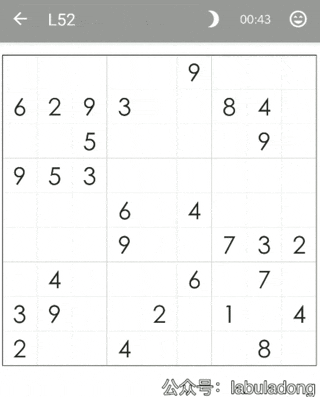Info

### # 一、直观感受Info

GIF 可能出现 bug，若卡住点开查看即可，下同。### # 二、代码实现

``````void solveSudoku(char[][] board);
``````

``````// 对 board[i][j] 进行穷举尝试
void backtrack(char[][] board, int i, int j) {
int m = 9, n = 9;
for (char ch = '1'; ch <= '9'; ch++) {
// 做选择
board[i][j] = ch;
// 继续穷举下一个
backtrack(board, i, j + 1);
// 撤销选择
board[i][j] = '.';
}
}
``````

emmm，再继续细化，并不是 1 到 9 都可以取到的，有的数字不是不满足数独的合法条件吗？而且现在只是给 `j` 加一，那如果 `j` 加到最后一列了，怎么办？

``````void backtrack(char[][] board, int i, int j) {
int m = 9, n = 9;
if (j == n) {
// 穷举到最后一列的话就换到下一行重新开始。
backtrack(board, i + 1, 0);
return;
}

// 如果该位置是预设的数字，不用我们操心
if (board[i][j] != '.') {
backtrack(board, i, j + 1);
return;
}

for (char ch = '1'; ch <= '9'; ch++) {
// 如果遇到不合法的数字，就跳过
if (!isValid(board, i, j, ch))
continue;

board[i][j] = ch;
backtrack(board, i, j + 1);
board[i][j] = '.';
}
}

// 判断 board[i][j] 是否可以填入 n
boolean isValid(char[][] board, int r, int c, char n) {
for (int i = 0; i < 9; i++) {
// 判断行是否存在重复
if (board[r][i] == n) return false;
// 判断列是否存在重复
if (board[i][c] == n) return false;
// 判断 3 x 3 方框是否存在重复
if (board[(r/3)*3 + i/3][(c/3)*3 + i%3] == n)
return false;
}
return true;
}
``````

emmm，现在基本上差不多了，还剩最后一个问题：这个算法没有 base case，永远不会停止递归。这个好办，什么时候结束递归？显然 `r == m` 的时候就说明穷举完了最后一行，完成了所有的穷举，就是 base case

``````boolean backtrack(char[][] board, int i, int j) {
int m = 9, n = 9;
if (j == n) {
// 穷举到最后一列的话就换到下一行重新开始。
return backtrack(board, i + 1, 0);
}
if (i == m) {
// 找到一个可行解，触发 base case
return true;
}

if (board[i][j] != '.') {
// 如果有预设数字，不用我们穷举
return backtrack(board, i, j + 1);
}

for (char ch = '1'; ch <= '9'; ch++) {
// 如果遇到不合法的数字，就跳过
if (!isValid(board, i, j, ch))
continue;

board[i][j] = ch;
// 如果找到一个可行解，立即结束
if (backtrack(board, i, j + 1)) {
return true;
}
board[i][j] = '.';
}
// 穷举完 1~9，依然没有找到可行解，此路不通
return false;
}

boolean isValid(char[][] board, int r, int c, char n) {
// 见上文
}
``````

### # 三、算法可视化

``````void setNum(Button b, char n) {
// 输入一个方格，将该方格设置为数字 n
}

void cancelNum(Button b) {
// 输入一个方格，将该方格上的数字撤销
}
``````

``````for (char ch = '1'; ch <= '9'; ch++) {
Button b = new Button(r, c);
// 做选择
setNum(b, ch);
board[i][j] = ch;
// 继续穷举下一个
backtrack(board, i, j + 1);
// 撤销选择
cancelNum(b);
board[i][j] = '.';
}
``````